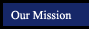Algebra 1 - Algebra 1 Concepts and SkillsChapter 1 Connections to Algebra Chapter 2 Properties of Real Numbers Chapter 3 Solving Linear Equations Chapter 4 Graphing Linear Equations and Functions Chapter 5 Writing Linear Equations Chapter 6 Solving and Graphing Linear Inequalities Chapter 7 Systems of Linear Equations and Inequalities Chapter 8 Exponents and Exponential Functions Chapter 9 Quadratic Equations and Functions Chapter 10 Polynomials and Factoring Chapter 11 Rational Expressions and Equations Chapter 12 Radicals and More Connections to Geometry

Notes for sections from AlgebraNotes.com